This is a standalone tutorial used in one of my courses. It introduces students to the Moran’s I analysis as well as some basic mapping features using `tmap`. This exercise also compares ArcMap’s Moran’s I results to those from a Monte Carlo simulation. For an overview of Moran’s I, see my lecture notes.

We will make use of the following packages: `sf` for importing the shapefiles, `tmap` for creating choropleth maps and `spdep` for implementing the Moran’s I analysis.

``````library(sf)
library(spdep)
library(tmap)``````

The following chunk loads the data (as an `sf` object) from the github site.

``````# Load the shapefile

If you want to load a shapefile from a local directory, simply use the `read_sf()` function as in

``````# Only run this chunk if you are loading a local shapefile

To list the column names associated with the object’s attribute table, type:

``names(s)``
`````` "NAME"       "STATE_NAME" "POP10_SQMI" "AVE_HH_SZ"  "AVE_FAM_SZ"
 "Income"     "House_year" "geometry"  ``````

To list the contents of an attribute, affix the dollar sign `\$` to the object name followed by the attribute name. For example, to list the income values, type:

``s\$Income``
``````  45765 59560 46559 50515 50027 40695 55046 43484 56701 60782 52393
 56139 55045 70906 65226 79368 59580 56851 37378 41665 45747 44543
 37110 39792 38239 42407``````

The Moran’s I statistic is not robust to outliers or strongly skewed datasets. It’s therefore good practice to check the distribution of the attribute values. You can plot the attribute values as follows:

``hist(s\$Income, main=NULL)``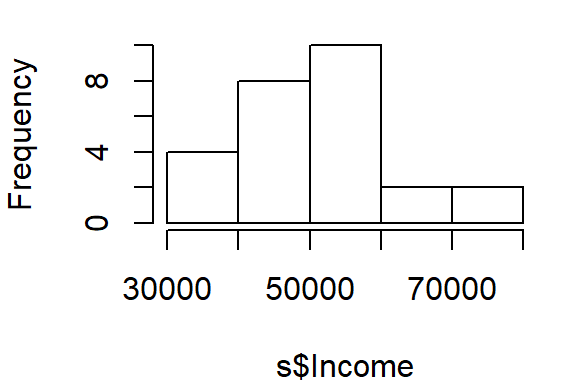or,

``boxplot(s\$Income, horizontal = TRUE)``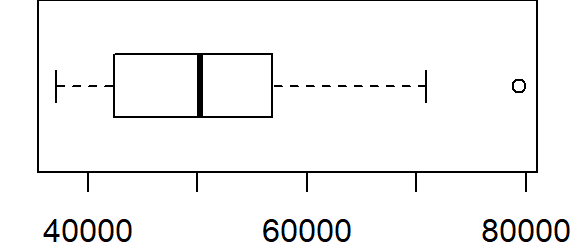Other than one outlier, the dataset seems to be well behaved. We’ll forgo any re-expression of the values going forward.

To symbolize the polygons using the `Income` attribute we will first define the classification breaks (`style = quantile` with `n = 8` breaks) and the symbol colors (`palette="Greens"`). For the latter, the `tmap` package makes use of Cynthia Brewer’s color schemes (see her website).

``````tm_shape(s) + tm_fill(col="Income", style="quantile", n=8, palette="Greens") +
tm_legend(outside=TRUE)``````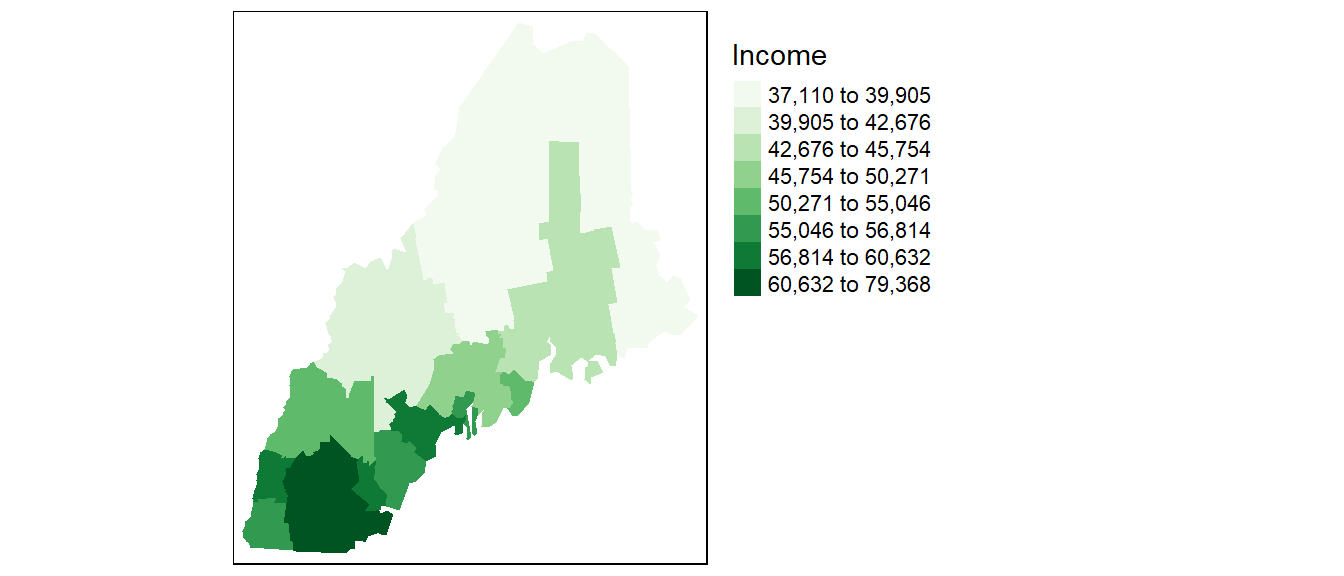## Moran’s I analysis

### Step 1: Define neighboring polygons

The first step in a Moran’s I analysis requires that we define “neighboring” polygons. This could refer to contiguous polygons, polygons within a certain distance, or it could be non-spatial in nature and defined by social, political or cultural “neighbors”.

Here, we’ll adopt a contiguous neighbor definition. We’ll accept any contiguous polygons that share at least one vertex; this is the “queen” case (if one chooses to adopt the chess analogy) and it’s parameterized as `queen = TRUE` in the call to `poly2nb`. If we required that just edges be shared between polygons then we would set `queen = FALSE` (the rook analogy).

``nb <- poly2nb(s, queen=TRUE)``

For each polygon in our shape object, `nb` lists all neighboring polygons. For example, to see the neighbors (by ID number) for the first polygon in the shape object, type:

``nb``
``````[]
  2  3  6  7 20``````

Here’s the list of county names and associated IDs:

County ID
Androscoggin 1
Cumberland 2
Kennebec 3
Knox 4
Lincoln 5
Oxford 6
Waldo 8
York 9
Belknap 10
Carroll 11
Cheshire 12
Grafton 13
Hillsborough 14
Merrimack 15
Rockingham 16
Strafford 17
Sullivan 18
Aroostook 19
Franklin 20
Hancock 21
Penobscot 22
Piscataquis 23
Somerset 24
Washington 25
Coos 26

### Step 2: Assign weights to the neighbors

Next, we need to assign weights to each neighboring polygon. In this example, each neighboring polygon will be assigned equal weight when computing the neighboring mean income values.

``lw <- nb2listw(nb, style="W", zero.policy=TRUE)``

To see the weight of the first polygon’s neighbors type:

``lw\$weights``
``````[]
 0.2 0.2 0.2 0.2 0.2``````

These are the weights each neighboring income value will be multiplied by before being summed. If a polygon has 5 neighbors, each neighbor will have a weight of 1/5 or 0.2. This weight will then be used to compute the mean neighbor values as in `0.2(neighbor1) + 0.2(neighbor2) + 0.2(neighbor3) + 0.2(neighbor4) + 0.2(neighbor5)`. This is equivalent to summing all five income values then dividing by 5.

### Step 3 (optional): Compute the (weighted) neighbor mean income values

NOTE: This step does not need to be performed when running the `moran` or `moran.test` functions outlined in Steps 4 and 5. This step is only needed if you wish to generate a scatter plot between the income values and their lagged counterpart.

Next, we’ll have R compute the average neighbor income value for each polygon. These values are often referred to as spatially lagged values.

``````inc.lag <- lag.listw(lw, s\$Income)
inc.lag``````
``````  48705.00 49551.75 45963.17 46755.50 48901.00 49748.50 50477.75
 46197.17 53057.00 58061.00 52535.00 63878.50 55531.80 64396.00
 63755.33 65237.33 62894.00 61829.00 39921.00 43202.75 42088.67
 40291.67 40571.00 41789.83 42556.00 49377.67``````

You can plot the relationship between income and its spatially lagged counterpart as follows. The fitted blue line added to the plot is the result of an OLS regression model.

``````plot(inc.lag ~ s\$Income, pch=16, asp=1)
M1 <- lm(inc.lag ~ s\$Income)
abline(M1, col="blue")``````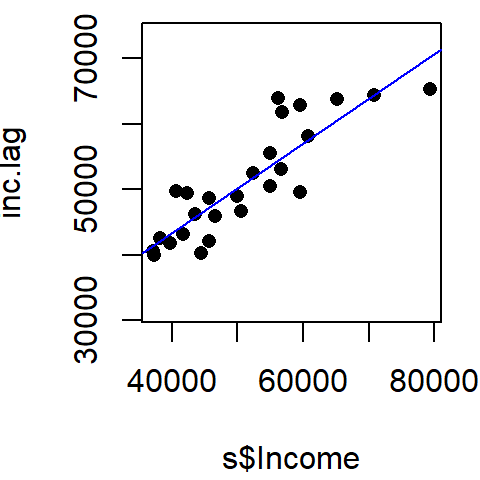The slope of the line is the Moran’s I coefficient. You can extract its value from the model object `M1` as follows:

``coef(M1)``
`````` s\$Income
0.6827955 ``````

The moran’s I coefficient is 0.68. The positive (upward) slope suggests that as the income value of a said polygon increases, so does those of its neighboring polygons. If the slope were negative (i.e. sloping downward), this would suggest a negative relationship whereby increasing values in a said polygon would be surrounded by polygons with decreasing income values.

### Step 4: Computing the Moran’s I statistic

The Moran’s I statistic can be computed using the `moran` function.

``````I <- moran(s\$Income, lw, length(nb), Szero(lw))
I``````
``````\$I
 0.6827955``````

Recall that the Moran’s `I` value is the slope of the line that best fits the relationship between neighboring income values and each polygon’s income in the dataset.

### Step 5: Performing a hypothesis test

The hypothesis we are testing states that “the income values are randomly distributed across counties following a completely random process”. There are two methods to testing this hypothesis: an analytical method and a Monte Carlo method. We’ll explore both approaches in the following examples.

#### Analytical method

To run the Moran’s I analysis using the analytical method, use the `moran.test` function.

``moran.test(s\$Income,lw, alternative="greater")``
``````
Moran I test under randomisation

data:  s\$Income
weights: lw

Moran I statistic standard deviate = 5.8525, p-value = 2.421e-09
alternative hypothesis: greater
sample estimates:
Moran I statistic       Expectation          Variance
0.68279551       -0.04000000        0.01525284 ``````

The Moran’s I statistic is 0.683 (same value that was computed using the `moran` function, as expected). The p-value is very small. Usually, when the p-value is very small it’s common practice to report it as `< 0.001`.

Note that ArcMap adopts this analytical approach to its hypothesis test however, it implements a two-sided test as opposed to the one-sided test adopted in the above example (i.e. `alternative = "greater"`). A two-sided p-value is nothing more than twice the one-sided p-value. Unfortunately, ArcMap does not seem to make this important distinction in any of its documentation. This distinction can have important ramifications as shown in the next example (Florida crime data). Fortunately, the income data is so strongly clustered that both a one-sided and two-sided test produce the same outcome (a p-value close to 0).

#### Monte Carlo method

The analytical approach to the Moran’s I analysis benefits from being fast. But it may be sensitive to irregularly distributed polygons. A safer approach to hypothesis testing is to run an MC simulation using the `moran.mc()` function. The `moran.mc` function takes an extra argument `n`, the number of simulations.

``````MC<- moran.mc(s\$Income, lw, nsim=999, alternative="greater")

# View results (including p-value)
MC``````
``````
Monte-Carlo simulation of Moran I

data:  s\$Income
weights: lw
number of simulations + 1: 1000

statistic = 0.6828, observed rank = 1000, p-value = 0.001
alternative hypothesis: greater``````

The MC simulation generates a very small p-value, 0.001. This is not surprising given that the income values are strongly clustered. We can see the results graphically by passing the Moran’s I model to the plot function:

``````# Plot the Null distribution (note that this is a density plot instead of a histogram)
plot(MC)``````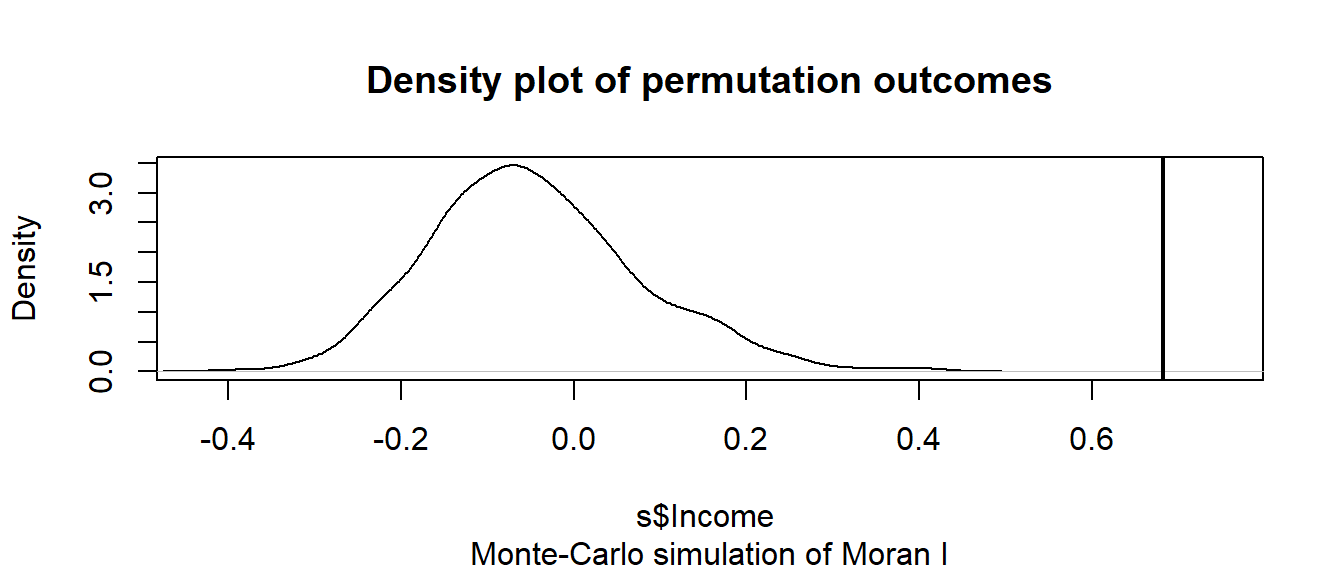The curve shows the distribution of Moran I values we could expect had the incomes been randomly distributed across the counties. Note that our observed statistic, 0.683, falls way to the right of the distribution suggesting that the income values are clustered (a positive Moran’s I value suggests clustering whereas a negative Moran’s I value suggests dispersion).

Now, had the Moran’s I statistic been negative (suggesting a dispersed pattern), you would probably want to set the `alternative` argument to `less` thus giving you the fraction of simulated `I` values more dispersed than your observed `I` value.

A visual exercise that you can perform to assess how “typical” or “atypical” your pattern may be relative to a randomly distributed pattern is to plot your observed pattern alongside a few simulated patterns generated under the null hypothesis.

``````set.seed(131)
s\$rand1 <- sample(s\$Income, length(s\$Income), replace = FALSE)
s\$rand2 <- sample(s\$Income, length(s\$Income), replace = FALSE)
s\$rand3 <- sample(s\$Income, length(s\$Income), replace = FALSE)

tm_shape(s) + tm_fill(col=c("Income", "rand1", "rand2", "rand3"),
style="quantile", n=8, palette="Greens", legend.show = FALSE) +
tm_facets( nrow=1)``````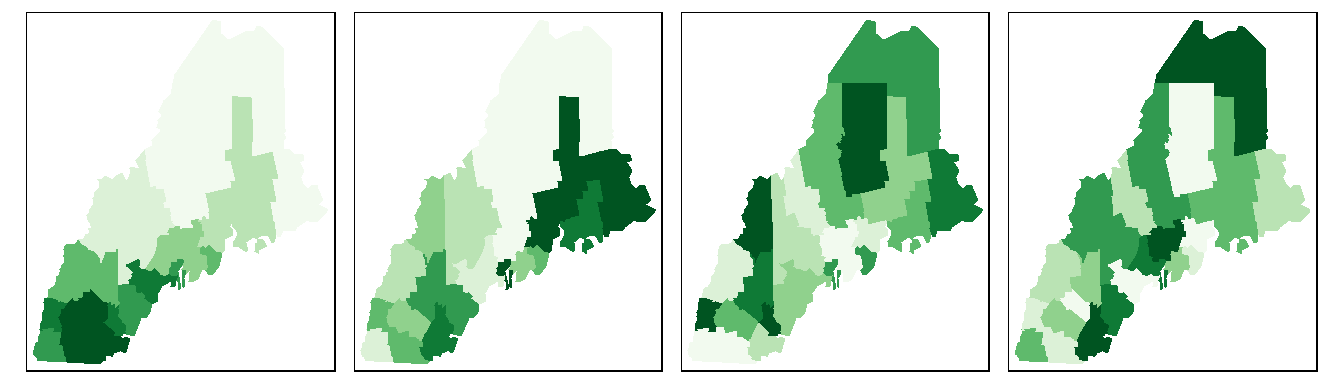Can you tell the difference between our observed income distribution and those generated from a completely random process? The map on the left is our observed distribution. The three maps on the right are realizations of a completely random process.

## Another example: Florida 1980 Homicide rate example

In this example, we explore the spatial distribution of 1980 homicide rates `HR80` by county for the state of Florida using the Monte Carlo approach.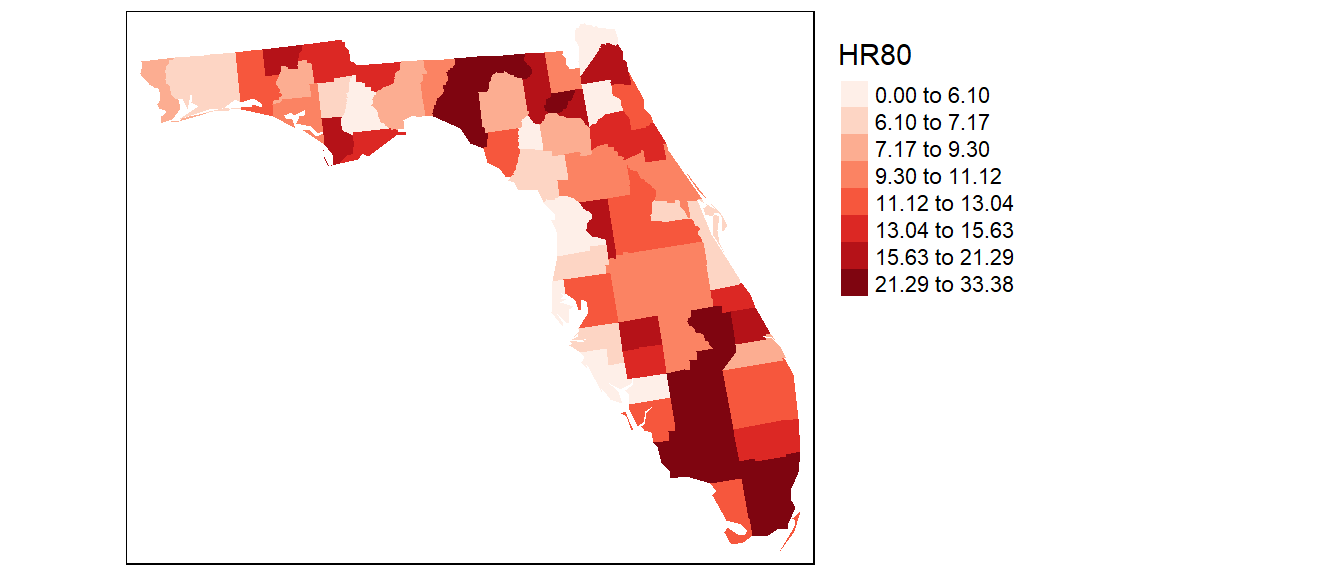The following code chunk highlights the entire workflow. Here, we’ll set the number of simulations to `9999`.

``````set.seed(2354)

# Define the neighbors (use queen case)
nb <- poly2nb(s, queen=TRUE)

# Compute the neighboring average homicide rates
lw <- nb2listw(nb, style="W", zero.policy=TRUE)

# Run the MC simulation version of the Moran's I test
M1 <- moran.mc(s\$HR80, lw, nsim=9999, alternative="greater")

# Plot the results
plot(M1)

# Display the resulting statistics
M1``````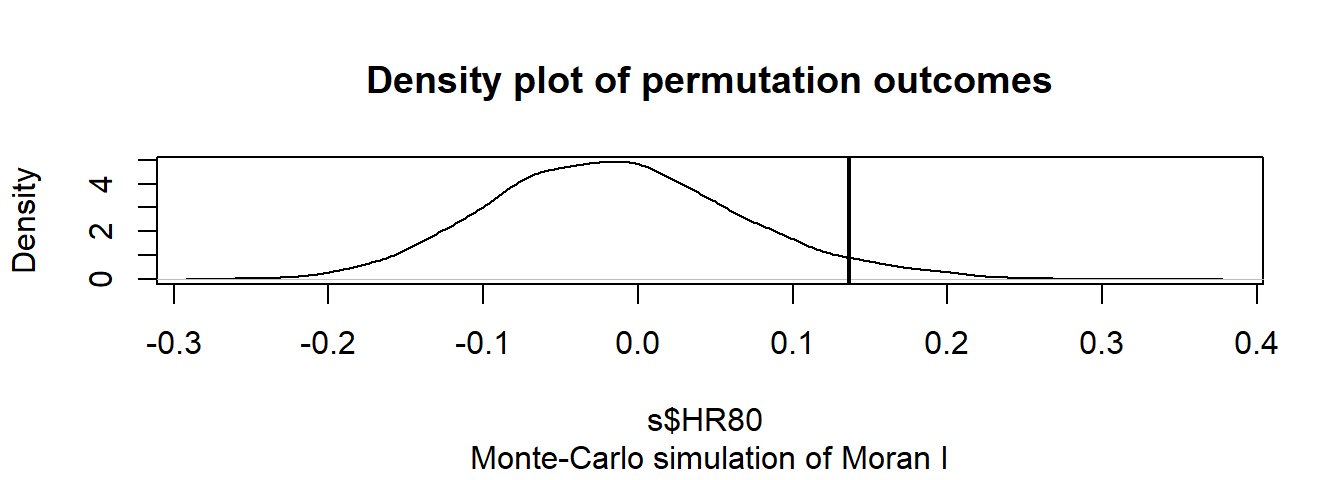``````
Monte-Carlo simulation of Moran I

data:  s\$HR80
weights: lw
number of simulations + 1: 10000

statistic = 0.13628, observed rank = 9575, p-value = 0.0425
alternative hypothesis: greater``````

The MC simulation generated a p-value of ~0.04 suggesting that there would be a ~4% chance of being wrong in rejecting the null hypothesis or that there is a ~4% chance that our observed pattern is consistent with a random process (note that your simulated p-value may differ from the one shown here–the number of simulations may need to be increased to reach a more stable convergence). Recall that this is a one-sided test. ArcMap’s analytical solution adopts a two-sided test. To compare its p-value to ours, we need to divide its p-value by 2 (i.e. `0.0588 / 2`) which gives us a one-sided p-value of `0.0294`–about 25% smaller than our simulated p-value.